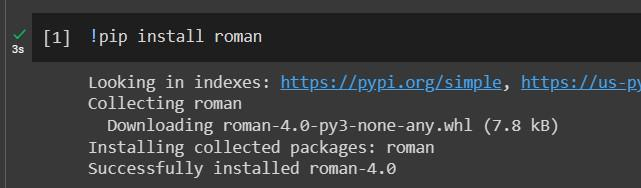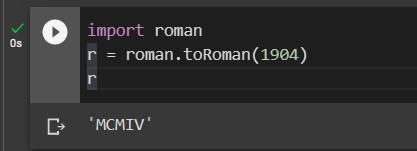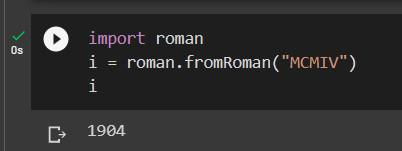GeeksforGeeks App
Open AppBrowser
Continue

# Python program to convert integer to roman

Given an integer, the task is to write a Python program to convert integer to roman.

Examples:

```Input: 5
Output: V

Input: 9
Output: IX

Input: 40
Output: XL

Input:  1904
Output: MCMIV```

Below table shows the list of Roman symbols including their corresponding integer values also:

Idea is to convert the units, tens, hundreds, and thousands of places of the given number separately. If the digit is 0, then there’s no corresponding Roman numeral symbol. The conversion of digits 4’s and 9’s are a little bit different from other digits because these digits follow subtractive notation.

Algorithm to convert an Integer value to Roman Numeral

Compare given number with base values in the order 1000, 900, 500, 400, 100, 90, 50, 40, 10, 9, 5, 4, 1. The base value that is just smaller or equal to the given number will be the initial base value (largest base value), Divide the number by its largest base value, the corresponding base symbol will be repeated quotient times, the remainder will then become the number for future division and repetitions. The process will be repeated until the number becomes zero.

## Method 1:

• Initially number = 3549, Since 3549 >= 1000 ; largest base value will be 1000 initially. And Divide 3549/1000. Quotient = 3, Remainder =549. The corresponding symbol M will be repeated thrice.
• Now, number become 549 and 1000 > 549 >= 500, largest base value will be 500 then divide 549/500. Quotient = 1, Remainder =49. The corresponding symbol D will be repeated once.
• Now, number = 49 and 50 > 49 >= 40, largest base value is 40. Then divide 49/40. Quotient = 1, Remainder = 9. The corresponding symbol XL will be repeated once.
• Now, number = 9 and 10> 9 >= 9, largest base value is 9. Then divide 9/9. Quotient = 1, Remainder = 0. The corresponding symbol IX will be repeated once.
• Finally, the number becomes 0, the algorithm stops here. The output obtained MMMDXLIX.

Below example shows the implementation of the above algorithm:

## Python3

 `# Python3 program to convert``# integer value to roman values` `# Function to convert integer to Roman values``def` `printRoman(number):``    ``num ``=` `[``1``, ``4``, ``5``, ``9``, ``10``, ``40``, ``50``, ``90``,``        ``100``, ``400``, ``500``, ``900``, ``1000``]``    ``sym ``=` `[``"I"``, ``"IV"``, ``"V"``, ``"IX"``, ``"X"``, ``"XL"``,``        ``"L"``, ``"XC"``, ``"C"``, ``"CD"``, ``"D"``, ``"CM"``, ``"M"``]``    ``i ``=` `12``    ` `    ``while` `number:``        ``div ``=` `number ``/``/` `num[i]``        ``number ``%``=` `num[i]` `        ``while` `div:``            ``print``(sym[i], end ``=` `"")``            ``div ``-``=` `1``        ``i ``-``=` `1` `# Driver code``if` `__name__ ``=``=` `"__main__"``:``    ``number ``=` `3549``    ``print``(``"Roman value is:"``, end ``=` `" "``)``    ``printRoman(number)`

Output:

`Roman value is: MMMDXLIX`

## Method 2:

In this method, we have to first observe the problem. The number given in the problem statement can be a maximum of 4 digits. The idea to solve this problem is:

• Divide the given number into digits at different places like one’s, two’s, hundred’s, or thousand’s.
• Starting from the thousand’s place print the corresponding roman value. For example, if the digit at thousand’s place is 3 then print the roman equivalent of 3000.
• Repeat the second step until we reach one’s place.

Suppose the input number is 3549. So, starting from thousand’s place we will start printing the roman equivalent. In this case, we will print in the order as given below:

• The Roman equivalent of 3000
• The Roman equivalent of 500
• The Roman equivalent of 40
• The Roman equivalent of 9

So, the output will be: MMMDXLIX

The below example shows the implementation of the above approach:

## Python3

 `# Python3 program for above approach` `# Function to calculate Roman values``def` `intToRoman(num):` `    ``# Storing roman values of digits from 0-9``    ``# when placed at different places``    ``m ``=` `["``", "``M``", "``MM``", "``MMM"]``    ``c ``=` `["``", "``C``", "``CC``", "``CCC``", "``CD``", "``D",``         ``"DC"``, ``"DCC"``, ``"DCCC"``, ``"CM "``]``    ``x ``=` `["``", "``X``", "``XX``", "``XXX``", "``XL``", "``L",``         ``"LX"``, ``"LXX"``, ``"LXXX"``, ``"XC"``]``    ``i ``=` `["``", "``I``", "``II``", "``III``", "``IV``", "``V",``         ``"VI"``, ``"VII"``, ``"VIII"``, ``"IX"``]` `    ``# Converting to roman``    ``thousands ``=` `m[num ``/``/` `1000``]``    ``hundreds ``=` `c[(num ``%` `1000``) ``/``/` `100``]``    ``tens ``=` `x[(num ``%` `100``) ``/``/` `10``]``    ``ones ``=` `i[num ``%` `10``]` `    ``ans ``=` `(thousands ``+` `hundreds ``+``           ``tens ``+` `ones)` `    ``return` `ans` `# Driver code``if` `__name__ ``=``=` `"__main__"``:``    ``number ``=` `3549``    ``print``(intToRoman(number))`

Output:

`MMMDXLIX`

## Method 3:

In this approach, we consider the main significant digit in the number. Ex: in 1234, the main significant digit is 1. Similarly, in 345 it is 3. In order to extract main significant digit out, we need to maintain a divisor (lets call it div) like 1000 for 1234 (since 1234 / 1000 = 1) and 100 for 345 (345 / 100 = 3). Also, let’s maintain a dictionary called roman numeral = {1 : ‘I’, 5: ‘V’, 10: ‘X’, 50: ‘L’, 100: ‘C’, 500: ‘D’, 1000: ‘M’}

The below example shows the implementation of the above algorithm:

## Python3

 `# Python 3 program to convert integer``# number to Roman values``import` `math` `def` `integerToRoman(A):``    ``romansDict ``=` `\``        ``{``            ``1``: ``"I"``,``            ``5``: ``"V"``,``            ``10``: ``"X"``,``            ``50``: ``"L"``,``            ``100``: ``"C"``,``            ``500``: ``"D"``,``            ``1000``: ``"M"``,``            ``5000``: ``"G"``,``            ``10000``: ``"H"``        ``}` `    ``div ``=` `1``    ``while` `A >``=` `div:``        ``div ``*``=` `10` `    ``div ``/``=` `10` `    ``res ``=` `""` `    ``while` `A:` `        ``# main significant digit extracted``        ``# into lastNum``        ``lastNum ``=` `int``(A ``/` `div)` `        ``if` `lastNum <``=` `3``:``            ``res ``+``=` `(romansDict[div] ``*` `lastNum)``        ``elif` `lastNum ``=``=` `4``:``            ``res ``+``=` `(romansDict[div] ``+``                        ``romansDict[div ``*` `5``])``        ``elif` `5` `<``=` `lastNum <``=` `8``:``            ``res ``+``=` `(romansDict[div ``*` `5``] ``+``            ``(romansDict[div] ``*` `(lastNum ``-` `5``)))``        ``elif` `lastNum ``=``=` `9``:``            ``res ``+``=` `(romansDict[div] ``+``                        ``romansDict[div ``*` `10``])` `        ``A ``=` `math.floor(A ``%` `div)``        ``div ``/``=` `10``        ` `    ``return` `res` `# Driver code``print``(``"Roman value for the integer is:"``                ``+` `str``(integerToRoman(``3549``)))`

Output:

`Roman value for the integer is: MMMDXLIX`

Time Complexity: O(n)

Auxiliary Space: O(n)

## Method – 4 –

In this approach we will use an external module called roman to convert an integer to roman and vice versa. We need to install it using pip command first. Write the below command in terminal.

`!pip install roman`Then we will use two methods , one to convert integer into roman another to convert roman into integer.

#### Converting Integer to Roman –

For this purpose we will use the toRoman() method of the roman package, it takes the integer value as it’s argument.

## Python3

 `# Importing the package``import` `roman` `# converting the integer to Roman``# and storing it in variable 'r'``r ``=` `roman.toRoman(``1904``)` `# Printing the converted value``print``(r)`

Output –

`MCMIV`Time Complexity – O(1)

Auxiliary Space – O(1)

#### Converting Roman to Integer –

Here we will convert a roman value to an integer value using fromRoman() method which takes the roman value as an argument, we need to pass that as a string.

## Python3

 `# Importing the module roman``import` `roman` `# Converting the roman value``# into integer and storing``# it in variable 'i'``i ``=` `roman.fromRoman(``"MCMIV"``)` `# Printing the converted value``print``(i)`

Output –

`1904`Time Complexity – O(1)

Auxiliary Space – O(1)

My Personal Notes arrow_drop_up